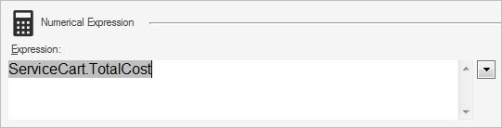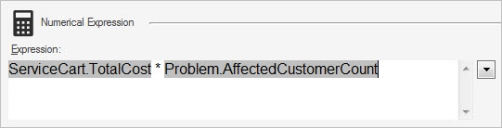# Define a Numerical Expression

A Numerical expression performs mathematical calculations using a string of tokens and mathematical symbols (example: Calculate the total cost of a Labor Cost Item by multiplying the work hours by the monetary rate).

While simple numbers can be used in an expression, only periods can be used as decimal separators to separate the integer from the fractional part of a number. Commas and other characters cannot be used in numerical expressions. Commas are not supported as decimal separators or as thousand separators (example: ten-thousand and five tenths should be entered as 10000.5). For more complex numerical formats, create a stored value for the number.

To define a Numerical expression:

1. Create an expression.
2. In the Editor drop-down list, select Number.
The Numerical Expression section opens.
3. Define the expression:
1. Select the Down arrow to open the Token Selector, and then select a value.
Right-click anywhere in the Expression field to access the Token Selector.
The token displays in the Expression field.2. Type a mathematical symbol for the equation (+ to add, - to subtract, * to multiply, or / to divide).3. Select the Down arrow to open the Token Selector, and then select a value.
Right-click anywhere in the Expression field to access the Token Selector.
The token displays in the Expression field.